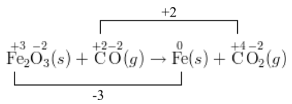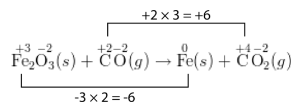# 22.9: Balancing Redox Reactions- Oxidation Number Change Method

$$\newcommand{\vecs}{\overset { \rightharpoonup} {\mathbf{#1}} }$$ $$\newcommand{\vecd}{\overset{-\!-\!\rightharpoonup}{\vphantom{a}\smash {#1}}}$$$$\newcommand{\id}{\mathrm{id}}$$ $$\newcommand{\Span}{\mathrm{span}}$$ $$\newcommand{\kernel}{\mathrm{null}\,}$$ $$\newcommand{\range}{\mathrm{range}\,}$$ $$\newcommand{\RealPart}{\mathrm{Re}}$$ $$\newcommand{\ImaginaryPart}{\mathrm{Im}}$$ $$\newcommand{\Argument}{\mathrm{Arg}}$$ $$\newcommand{\norm}{\| #1 \|}$$ $$\newcommand{\inner}{\langle #1, #2 \rangle}$$ $$\newcommand{\Span}{\mathrm{span}}$$ $$\newcommand{\id}{\mathrm{id}}$$ $$\newcommand{\Span}{\mathrm{span}}$$ $$\newcommand{\kernel}{\mathrm{null}\,}$$ $$\newcommand{\range}{\mathrm{range}\,}$$ $$\newcommand{\RealPart}{\mathrm{Re}}$$ $$\newcommand{\ImaginaryPart}{\mathrm{Im}}$$ $$\newcommand{\Argument}{\mathrm{Arg}}$$ $$\newcommand{\norm}{\| #1 \|}$$ $$\newcommand{\inner}{\langle #1, #2 \rangle}$$ $$\newcommand{\Span}{\mathrm{span}}$$$$\newcommand{\AA}{\unicode[.8,0]{x212B}}$$

Sulfuric acid is produced in extremely large quantities in the United States (about 40 million tons/year). This material is manufactured by oxidizing sulfur to form sulfur trioxide. The $$\ce{SO_3}$$ is then dissolved in water to form the sulfuric acid. Most of the sulfuric acid produced is used in fertilizers. This acid is also found in lead-acid car batteries.

## Balancing Redox Reactions: Oxidation-Number-Change Method

One way to balance redox reactions is by keeping track electron transfer, by using the oxidation numbers of each of the atoms. For the oxidation-number-change method, start with the unbalanced skeleton equation. The example below is for the reaction of iron (II) oxide with carbon monoxide. This reaction is one that takes place in a blast furnace during the processing of iron ore into metallic iron:

$\ce{Fe_2O_3} \left( s \right) + \ce{CO} \left( g \right) \rightarrow \ce{Fe} \left( s \right) + \ce{CO_2} \left( g \right)\nonumber$

$\overset{+3}{\ce{Fe_2}} \overset{-2}{\ce{O_3}} \left( s \right) + \overset{+2}{\ce{C}} \overset{-2}{\ce{O}} \left( g \right) \rightarrow \overset{0}{\ce{Fe}} \left( s \right) + \overset{+4}{\ce{C}} \overset{-2}{\ce{O_2}} \left( g \right)\nonumber$The carbon atom's oxidation number increases by 2, while the iron atom's oxidation number decreases by 3. As written, the number of electrons lost does not equal the number of electrons gained. In a balanced redox equation, these must be equal. So, the increase in oxidation number of one atom must be made equal to the decrease in oxidation number of the other.

Step 4: Use coefficients to make the total increase in oxidation number equal to the total decrease in oxidation number. In this case, the least common multiple of 2 and 3 is 6. So the oxidation-number increase should be multiplied by 3, while the oxidation-number decrease should be multiplied by 2. The coefficient is also applied to the formulas in the equation. So a 3 is placed in front of the $$\ce{CO}$$ and in front of the $$\ce{CO_2}$$. A 2 is placed in front of the $$\ce{Fe}$$ on the right side of the equation. The $$\ce{Fe_2O_3}$$ does not require a coefficient because the subscript of 2 after the $$\ce{Fe}$$ indicates that there are already two iron atoms.Step 5: Check the balancing for both atoms and charge. Occasionally, a coefficient may need to be placed in front of a molecular formula that was not involved in the redox process. In the current example, the equation is now balanced.

$\ce{Fe_2O_3} \left( s \right) + 3 \ce{CO} \left( g \right) \rightarrow 2 \ce{Fe} \left( s \right) + 3 \ce{CO_2} \left( g \right)\nonumber$Figure $$\PageIndex{1}$$: A blast furnace is where iron ore is processed and turned into iron metal. First, air is blown through a mixture of iron ore and coke (carbon). The carbon monoxide produced reduces the $$\ce{Fe^{3+}}$$ ions in the iron ore to metallic iron.

## Summary

This page titled 22.9: Balancing Redox Reactions- Oxidation Number Change Method is shared under a CK-12 license and was authored, remixed, and/or curated by CK-12 Foundation via source content that was edited to the style and standards of the LibreTexts platform; a detailed edit history is available upon request.